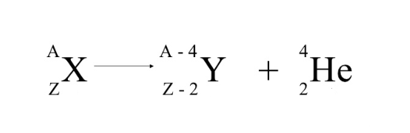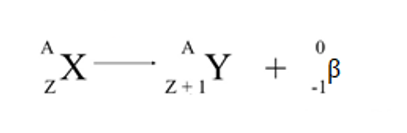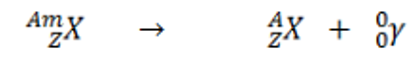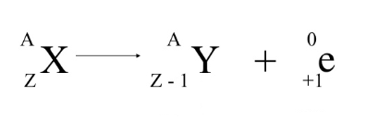# The equation below is best described as ___________. ²³⁵₉₂U → ²³⁵₉₃Np + ⁰₋₁eA) alpha decay.B) beta decay.C) gamma emission.D) positron emission.

Question
74 views
The equation below is best described as ___________. ²³⁵₉₂U → ²³⁵₉₃Np + ⁰₋₁e
A) alpha decay.
B) beta decay.
C) gamma emission.
D) positron emission.

check_circle

Step 1

Alpha decay is one process that unstable atoms can use to become more stable. During alpha decay, an atom's nucleus sheds two protons and two neutrons, called an alpha particle. This  results in the decrease of mass number (A) by 4 and also, the atomic number (Z) decreases by 2. It can be represented as:Beta decay (β-decay) is a type of radioactive decay in which a beta particle (fast energetic electron or positron) is emitted from an atomic nucleus.

During beta decay, a neutron is transformed into a proton by the emission of an electron, resulting in the same mass number (A) but the atomic number (Z) increases by 1. It can be represented as:Gamma decay, type of radioactivity in which some unstable atomic nuclei dissipate excess energy by a spontaneous electromagnetic process. In the most common form of gamma decay, known as gamma emission, gamma rays are radiated.

There is no change in the same mass number (A) or the atomic number (Z). It can be represented as:Positron emission or beta plus decay (β+ decay) is a type of radioactive decay in which a positron is emitted from an atomic nucleus.

During positron emission, a proton is transformed into a neutron and the excess positive charge is emitted, resulting in the same mass number (A) but the atomic number (Z) decreases by 1. It can be represented as:Step 2

Given,

To determine the type of decay for the following equation :

²³⁵₉₂U → ²³⁵₉₃Np + ⁰₋₁e

Mass number of U = 235

Atomic number of U = 92

Mass number of Np = 235

Atomic number of Np = 93

When U u...

### Want to see the full answer?

See Solution

#### Want to see this answer and more?

Solutions are written by subject experts who are available 24/7. Questions are typically answered within 1 hour.*

See Solution
*Response times may vary by subject and question.
Tagged in